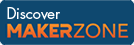Cody

# Problem 20. Summing digits

Created by Cody Team in Community

Given n, find the sum of the digits that make up 2^n.

Example:

``` Input  n = 7
Output b = 11```

since 2^7 = 128, and 1 + 2 + 8 = 11.

### Solution Stats

50.83% Correct | 49.17% Incorrect
Last solution submitted on Mar 26, 2019

#### TagsMATLAB and Simulink resources for Arduino, LEGO, and Raspberry Pi